LR  >  Alphanumeric Series, Introduction and Examples (with Solutions) - 1

# Alphanumeric Series, Introduction and Examples (with Solutions) - 1 | Reasoning Aptitude for Competitive Examinations - LR

 1 Crore+ students have signed up on EduRev. Have you?

Alphanumeric (sometimes shortened to alphameric) is a combination of alphabetic and numeric characters. In some cases, it may include upper and lower case letters, punctuation marks, and symbols (such as @, &, and *).
This type of reasoning is a mixed bag of coding-decoding, series-based reasoning and finding positions based reasoning questions.

Type of question

1. Immediately Preceded By
2. Immediately Followed By
3. Based on word/ Number / symbol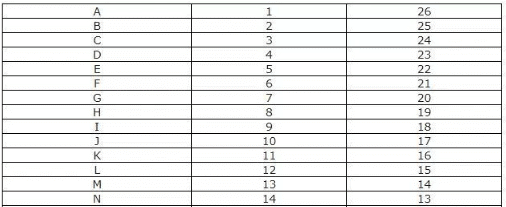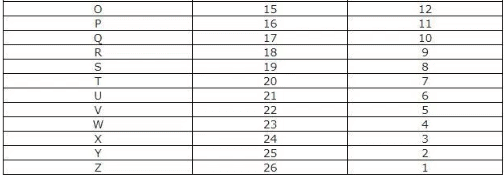Example 1: Study it carefully and answer the questions that follow:
L F 3 # R N 8 A @ Y 4 M © W P 6 H U 9 I K 2 E
Q. If all numbers are dropped from the above arrangement, which of the following will be the fourteenth from the left end?
(a) I
(b) P
(c) W
(d) U
(e) None of these

If all number are dropped, the arrangement will look like-
L F # R N A @ Y M © W P H U I K E
ATQ, 14th From Left end will be U-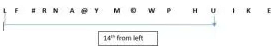Example 2: Study the following arrangement carefully and answer the questions given below.
R 4 P I J M Q 3 % T @ © U K 5 V 1 W \$ Y 2 B E 6 # 9 D H 8 G & Z N
Q. How many such numbers are there in the above arrangement each of which is immediately preceded by a vowel?
(a) One
(b) Two
(c) Three
(d) None of these

Step 1: 1st we have to identify all the vowel in the arrangement.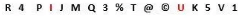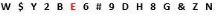Vowel in Above arrangement: I, U, E
Step 2: ATQ, numbers in the above arrangement each of which is immediately preceded by a vowel is________.
Since, we can see there is no such number which is preceded by vowel hence, the answer is none of these.

Example 3: C2E, E5H, G12K, 127N, ____?
(a) I58P
(b) J58Q
(c) K58Q
(d) I57Q

For Numbers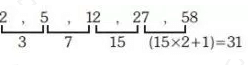For Alphabet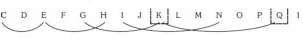So option (c) K58Q.

Practice Problems On Alphanumeric Series

Direction: Study the set of numbers given below and answer the questions given below:
247 851 389 725 598

Q.1. Which of the following numbers will be obtained if the first digit of the 2nd lowest number is subtracted from the second digit of the greatest number?
(a) 1
(b) 2
(c) 3
(d) 4
(e) 5

2nd lowest number is = 389 first digit of the 2nd lowest number is = 3
greatest number = 851
second digit of the greatest number = 5
subtraction = 5 – 3 = 2

Q.2. If in each number, first and the last digits are interchanged, which of the following will be the highest number?
(a) 247
(b) 851
(c) 389
(d) 725
(e) 598

247 851 389 725 598
742 158 983 527 895
So, 389 is the correct answer.

Q.3. If in each number the second and the third digits are interchanged, which will be the second lowest number?
(a) 247
(b) 851
(c) 389
(d) 725
(e) 598

247 851 389 725 298
274 815 398 752 589

Q.4. If one is subtracted from the last digit of each of the numbers and then the first and the third digits are interchanged, which of the following number will be the lowest?
(a) 247
(b) 851
(c) 389
(d) 725
(e) 598

247 851 389 725 598
642 158 983 527 895

Q.5. If in each number, all the three digits are arranged in ascending order, which of the following will be the highest number?
(a) 247
(b) 851
(c) 389
(d) 725
(e) 589

247 851 389 725 598
247 158 389 257 589

Directions: Study the following information carefully and answer the questions given beside:
578     796     429     685     849
Q.6. If all the digits are arranged in increasing order from left to right within the number then what would be the difference between second highest and second lowest number thus obtained?
(a) 75
(b) 89
(c) 94
(d) 97
(e) 99

The given sequence:
578     796     429     685     849
Arranging all the digits in increasing order from left to right within the number, we get:
578     679     249     568     489
Second highest number = 578
Second lowest number = 489
Required Difference = 578 – 489 = 89

Q.7. If first and last digit of each number is interchanged then what will the difference between the sum of even numbers and the sum of odd numbers thus obtained?
(a) 796
(b) 826
(c) 846
(d) 866
(e) 886

The given sequence:
578     796     429     685     849
Interchanging first and last digit of each number, we get
875     697     924     586     948
Sum of even numbers = 924 + 586 + 948 = 2458
Sum of odd numbers = 875 + 697 = 1572
Required Difference = 2458 – 1572 = 886

Q.8. If 142 is subtracted from each of the given numbers and the digits of each of these numbers are sumed up then which of the following numbers will have the highest sum thus obtained?
(a) 578
(b) 796
(c) 429
(d) 685
(e) 849

The given sequence:
578     796     429     685     849
Subtracting 142 from each number of the above sequence, we get:
436     654     287     543     707
After taking sum of the digits of each of the above numbers, we get
13     15     17     12     14
Here, the number which has the highest sum of digits is 429.

Q.9. If 2 is subtracted from each odd digit of each of the given numbers and 1 is added to each even digit of each of the given numbers in the above sequence then what is the difference between the least and the highest numbers?
(a) 576
(b) 488
(c) 510
(d) 598
(e) 618

The given sequence:
578     796     429     685     849
Subtracting 2 from each odd digit of each of the given even number of the above sequence and adding 1 to each even digit of each of the given odd number, we get
359     577     537     793     957
The least number: 359
The highest number: 957
The required difference = 957 - 359 = 598

Q.10. If all the digits within the number are to be added till a single-digit number is obtained then how many of these newly formed numbers will be even?
(a) One
(b) Two
(c) Three
(d) More than Three

The given sequence:
578     796     429     685     849
Adding all the digits within the number till a single digit number is obtained,
20    22    15    19    21
2    4     6      1      3
Here, the total even numbers in the above sequence (highlighted in red) are 3.

Directions: Study the following arrangements of numbers, symbols and letters carefully, follow the instructions and answer the questions.
8 K L 5 I R T 8 2 % U * 1 S V ^ W N M Z 2 7 \$ F ! B ¥ 0
Q.11. If all odd numbers are deleted in the series which will be the 8th element from right end?
(a) M
(b) 8
(c) 2
(d) Z
(e) None of these

According to the given series -
8 K L 5 I R T 8 2 % U * 1 S V ^ W N M Z 2 7 \$ F ! B ¥ 0
After deleting all the odd numbers then series will be 8 K l R T 8 2 % U * S V ^ W N Z 2 \$ F ! B ¥ 0 And the 8th element the right end is Z.

Q.12. After eliminating all the alphabet from the series which will be the 10th element from the left side?
(a) T
(b) %
(c) \$
(d) 7
(e) None of these

According to the given series -
8 K L 5 I R T 8 2 % U * 1 S V ^ W N M Z 2 7 \$ F ! B ¥ 0
After eliminating all the alphabets then the series will be
8 5 8 2 % * 1 ^ 2 7 \$ ! ¥ 0
And the 10th element from the left side is 7.

Q.13. If we add all the numbers in the given series then the result would be?
(a) 33
(b) 23
(c) 27
(d) 32
(e) None of these

According to the given series -
8 K L 5 I R T 8 2 % U * 1 S V ^ W N M Z 2 7 \$ F ! B ¥ 0 Addition of all the numbers of the given series is 33. (8+5+8+2+1+2+7+0)

Q.14. If all the digits in the series are multiplied with 3 and then all numbers added, the resultant is?
(a) 101
(b) 100
(c) 90
(d) 99
(e) None of these

According to the given series - 8 K L 5 I R T 8 2 % U * 1 S V ^ W N M Z 2 7 \$ F ! B ¥ 0 And after the digits in the series are multiplied with 3, resultant is 99 (8*3+5*3+8*3+2*3+1*3+2*3+7*3+0*3).

Q.15. After eliminating all the symbols from the given series what will be the 3rd element to the right of the 5th element from the left side?
(a) 8
(b) 2
(c) N
(d) Z
(e) None of these

According to the given series -
8 K L 5 I R T 8 2 % U * 1 S V ^ W N M Z 2 7 \$ F ! B ¥ 0
After eliminating all the symbols series will be
8 K L 5 I R T 8 2 U 1 S V W N M Z 7 F B 0
And the 3rd element to the right of the 5th element from the left side is 8.

Directions: Study the following arrangements of numbers, symbols and letters carefully, follow the instructions and answer the questions.
R % 4 ! C 7 B 1 ^ N 3 π C F © 8 S Ω 9 L Q X & G £ D & R 3 * O

Q.16. If all the consonants are dropped from the given series which will be the 6th from the right of the 2nd element from the left end?
(a) N
(b) ^
(c) π
(d) 8
(e) None of these

According to the given series -
R % 4 ! C 7 B 1 ^ N 3 π C F © 8 S Ω 9 L Q X & G £ D & R 3 * O
After dropping all the consonants series will be
% 4 ! 7 1 ^ 3 π © 8 Ω 9 & £ & 3 * O
And the 6th from the right of the 2nd element from the left end is π.

Q.17. How many symbols are there in arrangement, followed by an alphabet and preceded by a number?
(a) 4
(b) 5
(c) 2
(d) 0
(e) None of these

According to the given series -
% 4 ! C 7 B 1 ^ N 3 π C F © 8 S Ω 9 L Q X & G £ D & R 3 * O
And total symbols followed by an alphabet and preceded by a number is 4.
(4 ! C 1 ^ N 3 π C 3 * O)

Q.18. What is the difference between the number 6th right end and 13th from left end based on reversing the series from the right end?
(a) 8
(b) 4
(c) 2
(d) 8
(e) None of these

According to the given series -
R % 4 ! C 7 B 1 ^ N 3 π C F © 8 S Ω 9 L Q X & G £ D & R 3 * O
After reversing the series from right end -
O * 3 R & D £ G & X Q L 9 Ω S 8 © F C π 3 N ^ 1 B 7 C ! 4 % R
And the difference between the number 6th right end and 13th from left end is 9 – 7 = 2

Q.19. What is the sum of place value according to alphabet series of the element in given series 7th from left end and 8th from right end? (consider A = 1; B = 2; C = 3;...So on till Z = 26)
(a) 8
(b) 9
(c) 10
(d) 11
(e) None of these

According to the given series -
R % 4 ! C 7 B 1 ^ N 3 π C F © 8 S Ω 9 L Q X & G £ D & R 3 * O
And the sum of place value according to alphabet series of the element in given series 7th from left end and 8th from right end is 2 + 7 = 9.(as B = 2 ; G = 7)

Q.20. In the given arrangement how many symbols are there between B and D?
(a) 7
(b) 6
(c) 4
(d) 8
(e) None of these

According to the given series -
R % 4 ! C 7 B 1 ^ N 3 π C F © 8 S Ω 9 L Q X & G £ D & R 3 * O And the symbols between B and D is 6.

The document Alphanumeric Series, Introduction and Examples (with Solutions) - 1 | Reasoning Aptitude for Competitive Examinations - LR is a part of the LR Course Reasoning Aptitude for Competitive Examinations.
All you need of LR at this link: LR

## Reasoning Aptitude for Competitive Examinations

12 videos|20 docs|116 tests

## Reasoning Aptitude for Competitive Examinations

12 videos|20 docs|116 tests

Track your progress, build streaks, highlight & save important lessons and more!

,

,

,

,

,

,

,

,

,

,

,

,

,

,

,

,

,

,

,

,

,

,

,

,

;